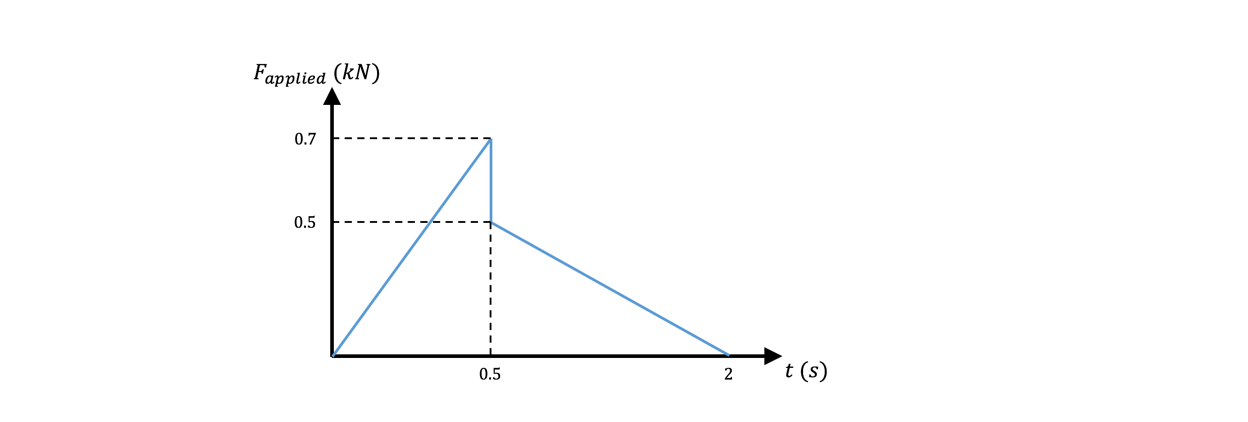# Problem: Consider the figure below, graphing the force applied on a 200 kg box vs time. During the first 0.5 s on the graph, the box remains at rest, while during the remaining time on the graph, the box moves at a constant speed of 15 m/s. What is the coefficient of static friction?

###### FREE Expert Solution
81% (149 ratings)
###### Problem Details

Consider the figure below, graphing the force applied on a 200 kg box vs time. During the first 0.5 s on the graph, the box remains at rest, while during the remaining time on the graph, the box moves at a constant speed of 15 m/s. What is the coefficient of static friction?